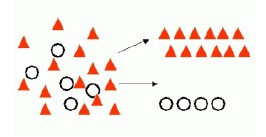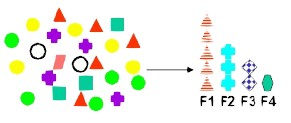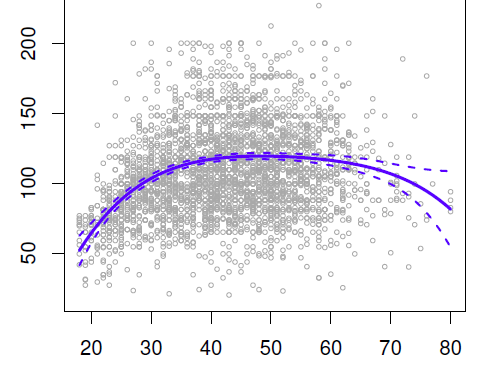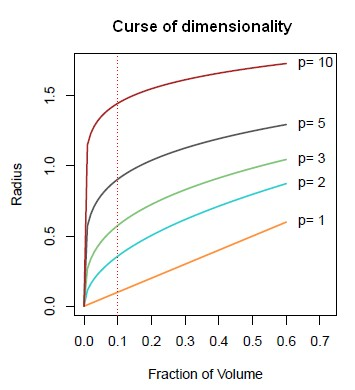# Machine Learning - Linear (Regression|Model)

Linear regression is a regression method (ie mathematical technique for predicting numeric outcome) based on the resolution of linear equation.

This is a classical statistical method dating back more than 2 centuries (from 1805).

The linear model is an important example of a parametric model.

Linear regression is very extensible and can be used to capture non-linear effects.

This is very simple model which means it can be interpreted.

There's, typically, a small number of coefficients. If we have a small number of features that are important,, it predicts future data quite well in a lot of cases, despite it's simplicity.

## Problem definition

You have a cloud of data points in (2|n) dimensions and are looking for the best straight (line|hyperline) fit.

You might have more than 2 dimensions. It's a standard matrix problem.

## Assumption

• Linear Regression assumes that the dependence of the target $Y$ on the predictors $X_1, ..., X_p$ is linear. Even if true regression functions are never linear.

## Model

It produces a model that is a linear function (i.e., a weighted sum) of the input attributes.

There are non-linear methods that build trees of linear models.

In two dimensions, it's a line, in three a plane, in N, a hyperplane.

Formula:

$Y = B_0 + B_1.X_1 + B_2.X_2+ \dots +B_p.X_p$

where:

Linear Regression works naturally with numeric classes (not with nominal ones) because the predictors are multiplied by weights but can be used for classification as well.

## Procedure

• Calculate weights (B) from training data. Large correlation is good, and the value cannot be greater than 1.
• Calculate an error (The squared error) on the training data and try to minimize it by choosing the best weights.

## Classification

### Two‐class problem

Linear regression can be used for binary classification as well:

• Calculate a linear function using regression
• and then apply a threshold to decide whether it's 0 or 1 (two-valued nominal classes).

Steps:

• On the training dataset: convert the class to binary attributes (0 and 1)
• Use the regression output and the nominal class as an input for One_Rule in order to define a threshold
• Use this threshold for predicting class 0 or 1

### Multi-class problem

For more class labels than 2, the following methods can be used:

• multi-response linear regression
• pairwise linear regression

Steps:

• Training: perform a regression for each class. N regression for a problem where there are n different classes. Set output to 1 for training instances that belong to the class, 0 for instances that don’t
• Prediction:
• choose the class with the largest output
• or use “pairwise linear regression”, which performs a regression for every pair of classes

Example for multi-response linear regression:
For a three class problem, we create three prediction model where the target is one class and zero for the others. If the actual and predicted outputs for the third instance are:

Instance Id Model Numeric Class Prediction
3 Blue 0 0.359
3 Green 1 0.322
3 Red 0 0.32

the predicted class is Blue because the first model predicts the largest output.

The actual class of the instance 3 is Green because the numeric class is a 1 in the second model

## Improvement

By replacing ordinary least squares fitting with some alternative fitting procedures, simple linear model can be improved in terms of:

• Prediction Accuracy: especially when p > n, to control the variance.

## Performance

M5P performs quite a lot better than Linear Regression.

## Implementation

### Weka

Weka has a supervised attribute filter (not the “unsupervised” one) called NominalToBinary that converts a nominal attribute into the same set of binary attributes used by LinearRegression and M5P.

To show the original instance numbers alongside the predictions, use the AddID unsupervised attribute filter, and the “Output additional attributes” option from the Classifier panel “More options …” menu. Be sure to use the attribute *index* (e.g., 1) rather than the attribute *name* (e.g., ID).

Recommended PagesData Mining - (Classifier|Classification Function)

A classifier is a Supervised function (machine learning tool) where the learned (target) attribute is categorical (“nominal”) in order to classify. It is used after the learning process to classify...Data Mining - (Discriminative|conditional) models

Discriminative models, also called conditional models, are a class of models used in machine learning for modeling the dependence of an unobserved variable y on an observed variable x. Discriminative...Data Mining - (Feature|Attribute) Extraction Function

Feature extraction is the second class of methods for dimension reduction. dimension reduction It creates new attributes (features) using linear combinations of the (original|existing) attributes. ...Data Mining - (Function|Model)

The model is the function, equation, algorithm that predicts an outcome value from one of several predictors. During the training process, the models are build. A model uses a logic and one of several...Data Mining - (Global) Polynomial Regression (Degree)

polynomials regression Although polynomials are easy to think of, splines are much better behaved and more local. With polynomial regression, you create new variables that are just transformations...Data Mining - (Stochastic) Gradient descent (SGD)

Gradient descent can be used to train various kinds of regression and classification models. It's an iterative process and therefore is well suited for map reduce process. The gradient descent update...Data Mining - Dimensionality (number of variable, parameter) (P)

Not to confound with d: the model size. You may have 1000 attributes (p=1000) in your sample but after feature selection for instance, you model may use only a handful (d=5) In physics and mathematics,...Data Mining - Elastic Net Model

In statistics and, in particular, in the fitting of linear or logistic regression models, the elastic net is a regularized regression method that linearly combines the L1 and L2 penalties of the lasso...Data Mining - Global vs Local

Global refers to calculation that are made over the whole data set whereas local refers to calculations that are made local to a point or a partition. In high dimension, it's really difficult to stay...Machine Learning - (Supervised|Directed) Learning ( Training ) (Problem)

Supervised Learning has the goal of predicting a value (outcome) from particular characteristics (predictors) that describes some behaviour. The attribute used to trained and being predicted is called...